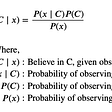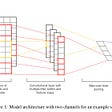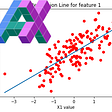# Linear Regression Using Least Squares| A High Schooler’s Guide To Deep Learning And AI

So now that you have some context as to what AI is and its history, we can begin jumping into the more interesting topics. In this article, I will show you how we can create our very first model: linear regression

Linear regression is the idea of computing a trend line for our data based on its parameters in order to predict the values of future data. For example, lets say that we want to create a model that predicts the SAT score of a student based on the amount of practice problems they do. If one of my friends asks me what their score could be assuming they did 1000 practice problems, I could compute a trend line based on prior data to find a rough estimate. One of the best ways to compute the trend line is the least-squares method.

Here is an example of what our model should be able to do when given points:

The importance of regression is that it defines the basic concepts behind neural networks. So, in order to understand the more complex structures that we will see, you need to first be able to interpret and replicate a simple regression model.

Let’s briefly look at the math and method behind our regression model. The least-squares method is a non-iterative method that creates the line of best fit for our data while trying to minimize the sum of the squares of the error that we receive from the formulas used. It will be responsible for computing our linear equation.

Assuming we have a set of n points: (x₁, y₁), (x₂, y₂),…, (xᵢ, yᵢ),…, (xn, yn)

We would need to find the line y = mx + b that fits these points as accurately as possible. Assuming that yᵢ is the actual value and f(xᵢ) is our predicted value, we use the following equation to find the error by taking the difference:

You’ll notice that the reason why the method we are using is called least-squares is because the equation above takes the square of the error function, and we are trying to minimize the error as much as possible. We also take the square, rather than taking the absolute value because it is simpler and allows us to compute the derivative, as shown below, much easier.

We can now use it to find m and b by taking the derivative with respect to m and b. First, let’s use it to find b:

We can use ȳ and to represent the mean values of all of the x and y coordinates in our data:

Then, we can say that b is:

And so, to find m, we would do:

Therefore:

The math seems scary on the surface level, but once you are able to comprehend what is happening, the entire process becomes trivial.

Next, let’s jump into the coding aspect of our models!

First, let’s import NumPy, our math library, and let’s implement the math that we just did in Python:

Once we run this, we should find that m is 0.26875 and b is ~1242.768. Feel free to try running this code with different numbers to see what you can find. Next, let’s import ‘matplotlib’, a library that allows us to plot our data:

When we run this, we should receive a plot of our data and a line of best fit that looks like this:

As you can see, we can now predict someone’s SAT score somewhat accurately based on the amount of problems they do. If more points were added into the data, we would see that our line of best fit becomes more and more accurate as it continues.

And we’re done! Wasn’t that super simple? Linear regression is incredibly easy to implement and it allows us to begin to understand how we can create more complex models. Although this is just the start, you’ll see that every project after this one is really just adding on to the basic regression model. In the next article, we will go over logistic regression in order to create even better predictions for data that may not be easily modeled with a linear equation. I hope to see you there!

--

--

--

High schooler interested in deep learning and artificial intelligence and wanting to teach others

Love podcasts or audiobooks? Learn on the go with our new app.

## Hello Datasphere — Towards a Systems Approach to Data Governance## Explore Tableau today if you’re working with data## Implementing Naïve Bayes Classification from Scratch with Python## Silica Nexus: глобальная онлайн-платформа, объединяющая видеоигры и пользователей 💎## Performing Analysis Of Meteorological Data## A summary of## Book connections within Project Gutenberg## Quitting work, saving babies and solving crimeHigh schooler interested in deep learning and artificial intelligence and wanting to teach others

## Machine Learning## Simple Linear Regression using Jax## The Ethical Data Scientist: Building Better Machine Learning Models## Decision Tree Algorithm